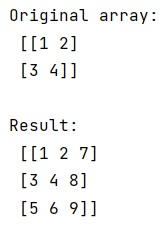# Simplest way to extend a NumPy array in 2 dimensions

Learn about the Simplest way to extend a NumPy array in 2 dimensions in Python?
Submitted by Pranit Sharma, on February 11, 2023

NumPy is an abbreviated form of Numerical Python. It is used for different types of scientific operations in python. Numpy is a vast library in python which is used for almost every kind of scientific or mathematical operation. It is itself an array which is a collection of various methods and functions for processing the arrays.

## Extending a NumPy array in 2 dimensions

Suppose that we are given a 2-dimensional numpy array and we need to find the most efficient way to add an extra row and column.

For this purpose, we can either use numpy.append() or numpy.vstack() and numpy.column_stack() methods to extend this multi-dimensional array.

Let us understand with the help of an example,

## Python code for the simplest way to extend a NumPy array in 2 dimensions

```# Import numpy
import numpy as np

# Creating a numpy array
arr =  np.array([[1,2],[3,4]])

# Display original array
print("Original array:\n",arr,"\n")

# Appending a row
arr = np.append(arr, [[5,6]], 0)

# Appending a column
arr = np.append(arr, [,,],1)

# Display result
print("Result:\n",arr)
```

Output: